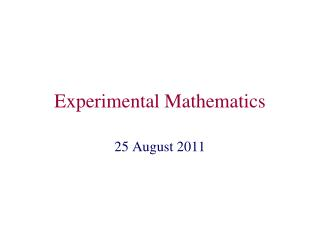DownloadDownload PresentationExperimental Mathematics

# Experimental Mathematics

Download Presentation## Experimental Mathematics

- - - - - - - - - - - - - - - - - - - - - - - - - - - E N D - - - - - - - - - - - - - - - - - - - - - - - - - - -
##### Presentation Transcript

1. Experimental Mathematics 25 August 2011

2. Linear Equations in 2D Space var('x,y') p=implicit_plot(2*x+3*y==5, (x,-10,10),(y,-10,10)) show(p)

3. Problem 1 Open a new Sage worksheet and do today’s problems within this worksheet. Find the intersection point of the lines determined by 4x+y=10 and 3x-4y=17 by a Sage plot. Hint: Two plots p,q can be combined by show(p+q)

4. Linear Equations in 3D Space var(‘x,y,z’) p=implicit_plot3d(2*x+3*y+5*z==-2, (x,-10,10),(y,-10,10),(z,-10,10),color=‘red’) show(p)

5. Problem 2 Combine the three planes determined by 2x+3y+5z=10 x-y+z=1 x+y-2z=0 in one plot. Plot each plane in a different color. Approximately identify the point where all three planes intersect.

6. Problem 3 The system x+y+z=-1 x-y+z=1 x+z=2 has no solution. Combine these three planes in one plot (with different colors) and find the “geometric reason” why there is no solution.

7. Vector and Matrix Operations in Sage v = vector([1,2]) w = vector([1,3]) A = matrix([[1,2],[2,3]]) B = matrix([[1,0,0],[1,1,2]]) A^3 A*B A*v 10*B 10*v def f(i,j): return i*j C = matrix(10,10,f) #2D vector #2D vector # 2x2 matrix # 2x3 matrix # matrix power # matrix product # matrix-vector product # scalar multiplication # scalar multiplication # C is 10x10 matrix with # C[i,j] = f(i,j)

8. Problem 4 • Try out matrix and vector computations as on the last slide. Note: vectors are different from lists and matrices are different from lists of lists. E.g. the append function does not work for vectors. b) Create the following matrix in Sage (where x is a symbolic variable):

9. Solving Linear Systems #Example: b = vector([1,2,2]) A = matrix([[1,2,-1],[2,3,1],[1,-1,0]]) x=A.solve_right(b) # solves Ax=b A*x # check

10. Problem 5 Use the solve_right function to solve the system from Problem 2: 2x+3y+5z=10 x-y+z=1 x+y-2z=0

11. Polynomial Interpolation

12. Example Find a polynomial of degree 2 through (0,4),(1,5),(2,5): # solve the linear system: x=vector([0,1,2]) y=vector([4,5,5]) n=2 def f(i,j): return x[i]^(n-j) A = matrix(3,3,f) coeffs = A.solve_right(y) #Compute polynomial and test: p(x) = 0 for i in range(3): p+=coeffs[i]*x^(n-i) print p #test: p(0); p(1); p(2)

13. Problem 6 a) Write a Python function Interpol(L) which returns a polynomial of degree n which passes through the n+1 points in the list L. For example, if L=[[0,0],[1,1],[2,4]], the function should return b) Apply your function to the example on the last slide and use it to find a polynomial of degree 5 through (0,4),(1,5),(2,5),(3,100),(4,-10),(5,-5).